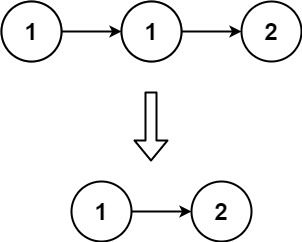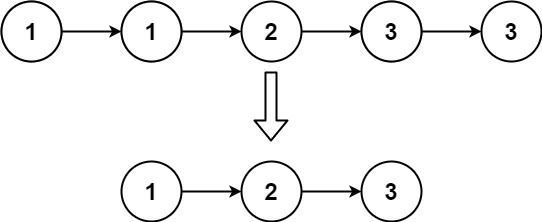# 83.remove-duplicates-from-sorted-list

## Statement``````输入：head = [1,1,2]

````````````输入：head = [1,1,2,3,3]

``````

• 链表中节点数目在范围 `[0, 300]`
• `-100 <= Node.val <= 100`
• 题目数据保证链表已经按升序 排列

Given the `head` of a sorted linked list, delete all duplicates such that each element appears only once. Return the linked list sorted as well.

Example 1:``````Input: head = [1,1,2]
Output: [1,2]
``````

Example 2:``````Input: head = [1,1,2,3,3]
Output: [1,2,3]
``````

Constraints:

• The number of nodes in the list is in the range `[0, 300]`.
• `-100 <= Node.val <= 100`
• The list is guaranteed to be sorted in ascending order.

## Solution

``````# Definition for singly-linked list.
# class ListNode:
#     def __init__(self, val=0, next=None):
#         self.val = val
#         self.next = next
class Solution:
def deleteDuplicates(self, head: ListNode) -> ListNode:
res = rt